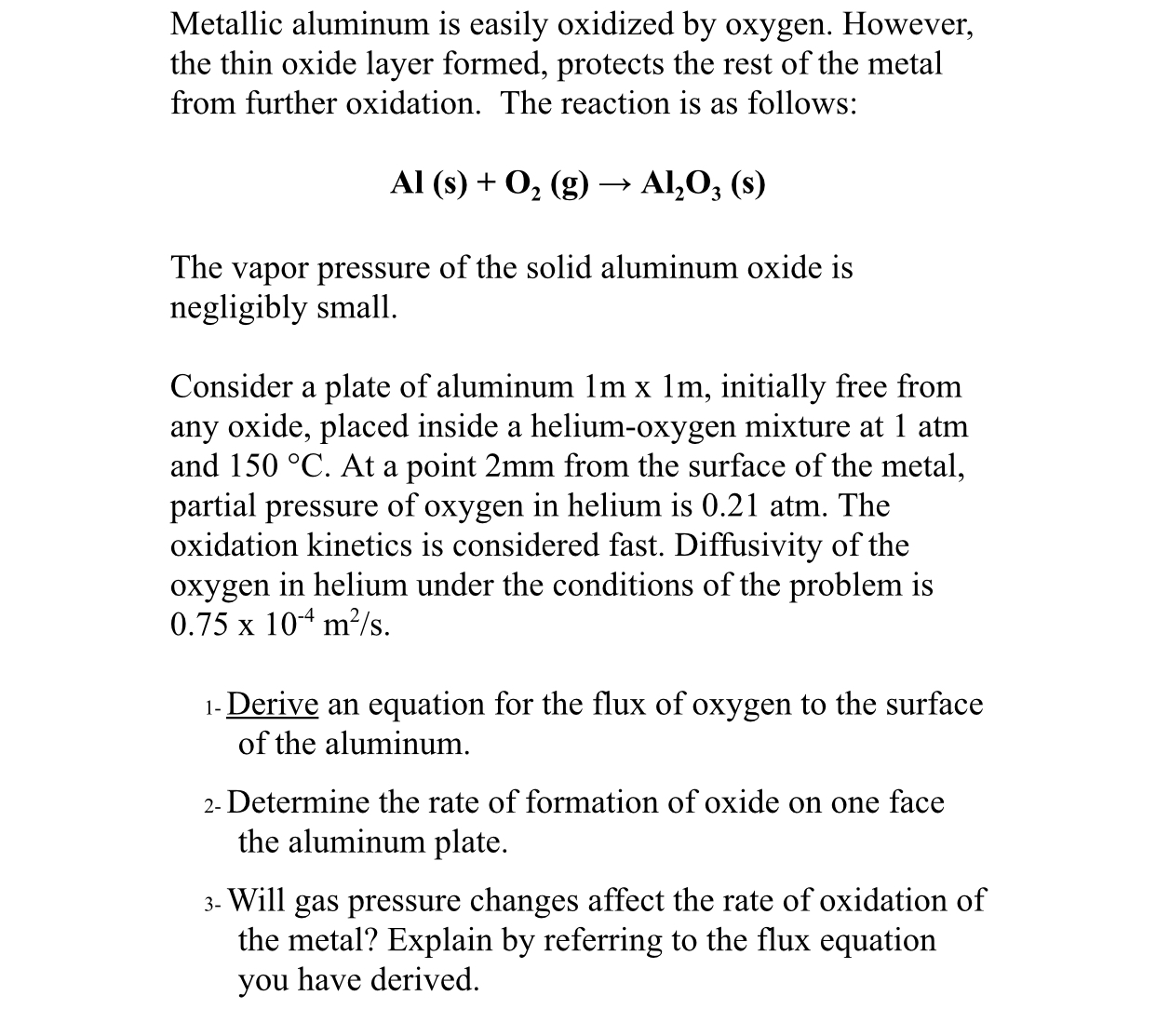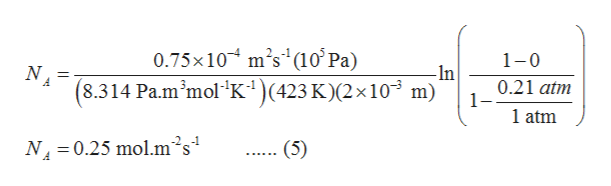# Metallic aluminum is easily oxidized by oxygen. However,the thin oxide layer formed, protects the rest of the metalfrom further oxidation. The reaction is as follows:Al (s)02 (g)> Al203 (s)The vapor pressure of the solid aluminum oxide isnegligibly smallConsider a plate of aluminum 1m x 1m, initially free fromany oxide, placed inside a helium-oxygen mixture at 1 atmand 150 °C. At a point 2mm from the surface of the metal,partial pressure of oxygen in helium is 0.21 atm. Theoxidation kinetics is considered fast. Diffusivity of theoxygen in helium under the conditions of the problem is0.75 x 104 m2/s1- Derive an equation for the flux of oxygen to the surfaceof the aluminum2- Determine the rate of formation of oxide on one facethe aluminum plate3- Will gas pressure changes affect the rate of oxidation ofthe metal? Explain by referring to the flux equationyou have derived

Questionhelp_outlineImage TranscriptioncloseMetallic aluminum is easily oxidized by oxygen. However, the thin oxide layer formed, protects the rest of the metal from further oxidation. The reaction is as follows: Al (s)02 (g) > Al203 (s) The vapor pressure of the solid aluminum oxide is negligibly small Consider a plate of aluminum 1m x 1m, initially free from any oxide, placed inside a helium-oxygen mixture at 1 atm and 150 °C. At a point 2mm from the surface of the metal, partial pressure of oxygen in helium is 0.21 atm. The oxidation kinetics is considered fast. Diffusivity of the oxygen in helium under the conditions of the problem is 0.75 x 104 m2/s 1- Derive an equation for the flux of oxygen to the surface of the aluminum 2- Determine the rate of formation of oxide on one face the aluminum plate 3- Will gas pressure changes affect the rate of oxidation of the metal? Explain by referring to the flux equation you have derived fullscreen
check_circleExpert Solution
Step 1

Let NA be the molar flux of oxygen (A) from the oxygen(A)-helium(C) mixture to the surface of the aluminum.

The Aluminum (B) is a non-diffusing component. The molar flux of aluminum in the given mixture is taken as zero ( N­B=0 ).

Consider the diffusion is steady-state diffusion and temperature and total pressure of the system is constant.

Step 2

(1)

The governing equation for the molar flux of component ‘A’ is represented by equation (1). Substituting the values of flux JA obtained from Fick's first law and mole fraction in partial pressures from Raoult's law gives equation (2).

Location 1 is at a distance of 2 mm from the surface of the metal which is at location 2.

Integrate the equation (2) from location 1 to location 2 with pA1 and pA2 as partial pressures of oxygen at 1 and 2.

The molar flux of oxygen to the surface of the aluminum is represented by equation (4)

Step 3

The reaction is given as an instantaneous reaction. As soon as the oxygen reaches the metal, it oxidizes the met...help_outlineImage Transcriptionclose0.75x10 m's (10' Pa) (8.314 Pa.m'mol K) (423 K)(2 x 10 m) 1-0 N -In 0.21 atm 1- 1 atm N4 0.25 molm's (5) fullscreen

### Want to see the full answer?

See Solution

#### Want to see this answer and more?

Solutions are written by subject experts who are available 24/7. Questions are typically answered within 1 hour*

See Solution
*Response times may vary by subject and question
Tagged in

### Mass Transfer